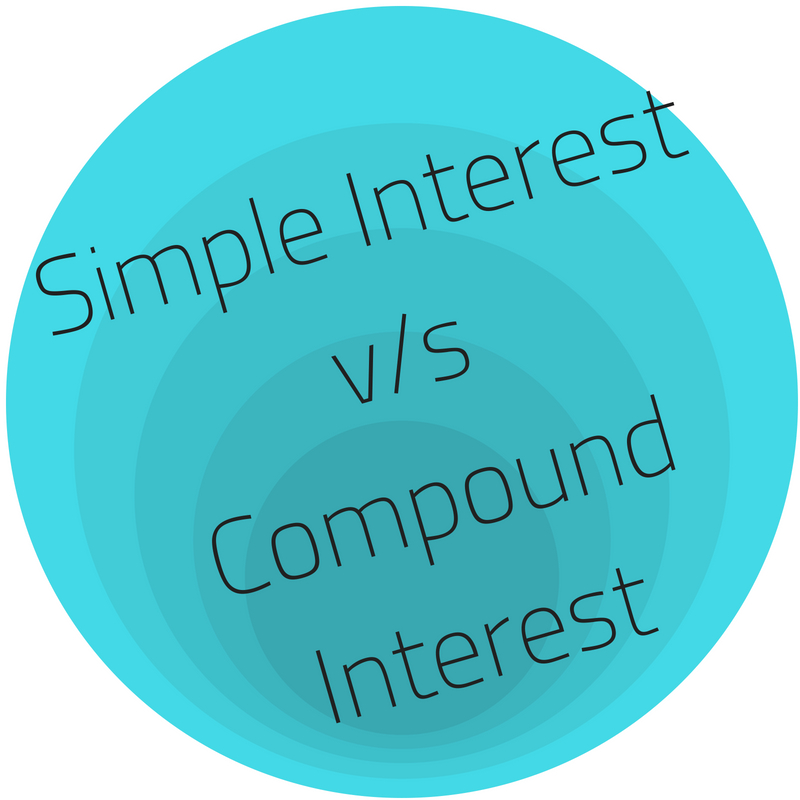# Simple Interest v/s Compound Interest: Exploring MoreWe have covered a fairly informative article on the topic of simple vs compound interest. However, this small mathematics wonder is not enough to be absorbed in just one reading. Hence, in this article, we are again re-emphasize on the topic and explain the same in a more vivid manner.

Both these words sound very familiar to us. Our childhood mathematics sessions have passed learning about simple interest and compound interest, but it important and very crucial to recall the meaning and difference between both, as both are highly useful in our everyday transactions. We may not realize it but our everyday life involves many transactions that have the application of simple and compound interest.

## Meaning

Let us first understand what does interest mean. Interest in financial terms means the cost of borrowing money, where the borrower pays a fee to the lender for using the latter’s money. Interest is expressed in terms of percentage that is calculated on a specific amount and can be classified as two types namely simple interest and compound interest.

It is pretty suggestive from the name “SIMPLE” interest that calculating such type of interest is very simple. Simple interest is the interest that one earns on their principal amount lent or kept somewhere. It is easier to compute simple interest as compared to compound interest. Conversely, compound interest refers to the interest that accrues on the principal amount as well as the accumulated interest of previous periods, which means it is interest on the principal amount as well as interest over interest.

When in a situation where one has to pay interest then there could be a vast difference in the amount of interest payable on a loan if interest is calculated on a compound basis rather than simple basis. In such a situation, simple interest works in your favor. On the contrary, while earning interest on your investments, the magic of compounding can work to your advantage and can be a powerful factor in wealth creation.

## Formulas

The formula for calculating simple interest is:

Simple Interest = Principal Amount x Interest Rate x Term of the loan

Or, SI=P X R X T

Additionally, the formula to calculate compound interest is:

Compound Interest = Total amount of Principal plus the Interest that we will earn in future minus the Principal amount at present

Compound Interest = Principal Amount X {(1 + Interest Rate) to the power of the number of compounding periods for a year} – the Principal amount at present

Or, CI = [P (1 + i)^n] – P

= P [(1 + i)^n– 1]

## Application

Both the concepts can be learned with the help of an example:

Say simple interest is to be charged at 5% on a sum of Rs.10,000 loan that is taken for a period of three-year , the total amount of simple interest payable by the borrower on this can be calculated as Rs.10,000 x 0.05 x 3 = Rs.1,500.

Interest on this loan is payable at Rs.500 annually (i.e. Rs.1,500/3), or Rs.1,500 over the three-year loan term.

On the other for the same example, if we want to calculate compound interest on the same example then, it would be: Rs. 10,000 [(1 + 0.05)3 – 1] ; = Rs.10,000 [1.157625 – 1] = Rs. 1,576.25 over the three-year loan term.

## Simple Interest v/s Compound Interest

Understanding the difference between simple and compound interest is vital when one is trying to pick the right loan or the right investment or even find the best place to store their savings. While both the types of interest will grow your money over time but there is a vast difference between the two.

If you consider things from a borrower’s perspective, who doesn’t want to get held with an expensive debt that takes years to eliminate, then probably they will want a loan with the type of interest that doesn’t compound. This is because with compounding interest, one has to consider the interest being added to the outstanding principal balance and the interest accumulated over the previous periods.

But from an investor’s point of view, looking to earn money, it’s best to search for an account with interest that compounds frequently. Compound interest has an edge over simple interest since it allows funds to grow at a faster rate than they would in an account with a simple interest rate. It comes into picture when one is calculating the annual percentage yield. The extra money that can be earned by compounding interest makes it the obvious preferred method over simple interest while earning money.

## Examples from real life situations

Compared to compound interest, simple interest is much easier to calculate and interpret. In real life situations, compound interest is the typical method applied in business situations, investments and financial products intended to run over multiple periods or years. Simple interest is mainly used for easy calculations and generally those which are for a single period or less than a year, though they also apply to open-ended situations, such as credit card balances, mortgages and most car loans.

## Conclusion

Familiarization with the basic concepts of simple and compound interest is very important as it helps in making better and thoughtful financial decisions, thus having a critical impact on our savings and investment decisions. Application of simple and compound interest is important to estimate future earnings and is vital for carrying out financial planning. Nowadays there are simple interest and compound interest calculators available online for people’s help. These can be used before taking up any investment or loan opportunity. In simpler words we can safely say that there is one simple rule: simple interest grows slowly, compounding speeds up the process and accordingly we can consider our investment or loaning opportunity.

## Related Posts